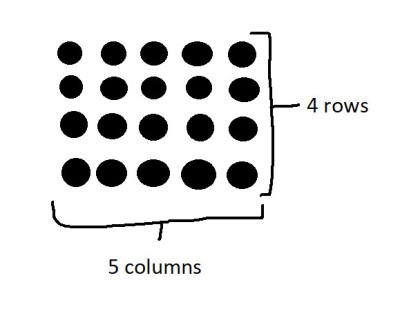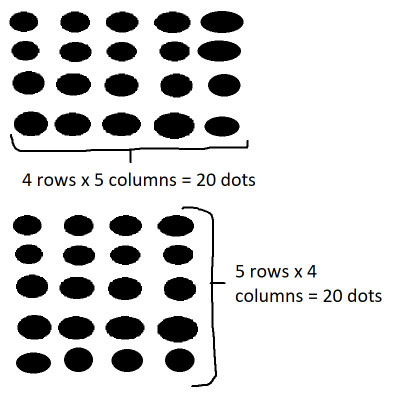# What Is Multiplication? (3 Key Things To Know About It)

Multiplication is important as part of a basic arithmetic skill set.  It is used in many areas, from finance to scientific research – but what does it really mean?

So, what is multiplication?  Multiplication is a shortcut for repeated addition. When you multiply two numbers A and B (factors), you are adding A to itself B times (or adding B to itself A times). A good way to visualize this is with dots, arranged into A rows and B columns. The total number of dots will be the product A*B.

Of course, there are some rules for multiplication that you should know (such as what happens when you multiply by zero, or when you multiply two negatives together).

In this article, we’ll talk about multiplication, provide a basic multiplication table, and look at some of the rules for multiplication that you should know.

Let’s get started.

## What Is Multiplication?

Multiplication is a shortcut for repeated addition.  It helps us to save time when performing calculations.

When you multiply two numbers A and B (which are called factors), you are adding A to itself B times:

• A*B = (A + A + A + … + A)  [repeated addition with B addends; that is, A appears in the sum B times]

Alternatively, you can think of it as adding B to itself A times (since multiplication is commutative – more on this later):

• A*B = (B + B + B + … + B)  [repeated addition with A addends; that is, B appears in the sum A times]

A good way to visualize the concept of multiplication is with dots, arranged into A rows and B columns. The total number of dots will be the product A*B.

For example, if you want to multiply 4*5 (which is 20), you would arrange your dots into 4 rows and 5 columns, as illustrated below:When we multiply 4 by 5, we get a product of 20. We can visualize this as 20 dots arranged into 4 rows and 5 columns.

You can also multiply 3 or more numbers together.  A good way to visualize multiplication of 3 numbers is a 3D “tower” of dots.

For the product A*B*C, the tower would have A rows, B columns, and C layers of dots.

For multiplication of 3 or more numbers, all you need to do is complete one multiplication at a time, going from left to right.

So:

• 2*3*4
• =6*4 [since 2*3 = 6]
• =24

Likewise:

• 2*3*4*5
• =6*4*5 [since 2*3 = 6]
• =24*5 [since 6*4 = 24]
• =120

If you memorize a multiplication table, you can improve your calculation speed and avoid counting on your fingers.

### Multiplication Table

Below is a multiplication table with numbers up to 12 for both factors.

If you memorize the table above, you will have a good start on learning how to do multiplication in your head.

The table might seem overwhelming at first, since there are 12*12 = 144 entries!  However, if you learn 4 entries per day, then it will only take you 36 days (just over 5 weeks) to learn them all by heart.

### What Are The Rules Of Multiplication?

There are several rules of multiplication to be aware of, including:

• Zero factor rule
• Zero product rule
• Factor of 1 rule
• Commutativity
• Associativity
• Signs of factors & products (positive & negative)
• Multiplication by powers of 10

Let’s take a closer look at each rule, starting with zero factors.

#### Zero Factor Rule

If either factor in multiplication is zero, then the product is zero.  In other words, zero times any number is zero:

• A*B = 0 if A = 0 (for any value of B)
• A*B = 0 if B = 0 (for any value of A)

Of course, this also means that 0*0 = 0.

Remember: no matter how many numbers you multiply together, if one of them is zero, then the product is zero.

So 1*2*3*4*5*6*0 is still zero.

#### Zero Product Rule

The zero product rule is sort of the “reverse” of the zero factor rule above.  If the product of multiplication is zero, then at least one of the factors is zero:

• If A*B = 0, then either A = 0 or B = 0 (or both are zero)

Remember: no matter how many numbers you multiply together, if the product is zero, then at least one of the numbers is zero.

So if a*b*c*d*e*f*g = 0, then at least one of a, b, c, d, e, f, or g is zero.

#### Factor Of 1 Rule

For a factor of 1 in multiplication, the product is the other factor.  In other words, 1 times a number is the number itself:

• 1*B = B (for any value of B)
• A*1 = A (for any value of A)

Of course, this also means that 1*1 = 1.

Remember: no matter how many 1s you multiply together, the product is 1.

So 1*1*1*1*1*1 is still 1.

#### Commutativity

Remember that multiplication is commutative.  In other words, the order of the factors does not matter.

So:

• A*B = B*A  [for any values of A and B]

Going back to the “rows and columns of dots” analogy, the way to think of this “visually” is as follows.  You will get the same number of dots (A*B) from both of these scenarios:

• A rows of B dots
• B rows of A dotsWhen we multiply 4 by 5 (or 5 by 4), we get a product of 20. We can visualize this as 20 dots arranged into 4 rows and 5 columns (or 5 rows and 4 columns).

The same rule applies for three or more factors.  For instance, with three factors A, B, and C, all six of the following expressions are equivalent:

• A*B*C
• A*C*B
• B*A*C
• B*C*A
• C*A*B
• C*B*A

#### Associativity

Remember that multiplication is associative.  In other words, the grouping of the factors does not matter.

So, we can perform any multiplication first and the result will be the same:

• (A*B)*C = A*(B*C)

Visually, these two items would have the same number of dots:

• a 3D tower of C layers, with each layer having A rows and B columns
• a 3D tower of A layers, with each layer having B rows and C columns

#### Signs Of Factors & Products (Positive & Negative)

When we multiply two factors together, the product is positive if both factors have the same sign, and negative otherwise.  That is:

• If we multiply two positive numbers, the product is positive.
• If we multiply two negative numbers, the product is positive (negatives “cancel” each other out to give us a positive).
• If we multiply a positive number and a negative number, the product is negative.

The following table summarizes the scenarios:

So:

• 2*3 = 6
• 2*(-3) = -6
• (-2)*3 = -6
• (-2)*(-3) = 6

We can apply similar reasoning for a product 3 or more factors.

If there are an even number of negative factors, then the product is positive.  Otherwise, the product is negative.

So:

• 2*3*4 = 24
• 2*3*(-4) = -24
• 2*(-3)*4 = -24
• 2*(-3)*(-4) = 24
• (-2)*3*4 = -24
• (-2)*3*(-4) = 24
• (-2)*(-3)*4 = 24
• (-2)*(-3)*(-4) = -24

#### Multiplication By Powers Of 10

If you multiply a number A by a power of 10, you can simply append zeros to the end of the number A to get the product.  Specifically, when you multiply A by 10N, append N zeroes to the end of A to get the product.

For example:

• 5*10 = 50 (A = 5, N = 1, so write A with an extra zero appended at the end)
• 16*100 = 1600 (A = 16, N = 2, so write A with two extra zeroes appended at the end)
• 25*1,000 = 25,000 (A = 25, N = 3, so write A with three extra zeroes appended at the end)
• 3*10,000 = 30,00 (A = 3, N = 4, so write A with four extra zeroes appended at the end)

## Conclusion

Now you know what multiplication is and what it means.  You also know how to visualize it, along with some of the important rules to remember.

There are lots of applications of multiplication in math, such as finding the area of a square.